Python | Pandas dataframe.reindex_like()

• Last Updated : 22 Nov, 2018

Python is a great language for doing data analysis, primarily because of the fantastic ecosystem of data-centric python packages. Pandas is one of those packages and makes importing and analyzing data much easier.

Pandas dataframe.reindex_like() function return an object with matching indices to myself. Any non-matching indexes are filled with NaN values.

Syntax:
Syntax : DataFrame.reindex_like(other, method=None, copy=True, limit=None, tolerance=None)

Parameters :
other : Object
method : string or None
copy : boolean, default True
limit : Maximum number of consecutive labels to fill for inexact matches.
tolerance : Maximum distance between labels of the other object and this object for inexact matches. Can be list-like.

Returns : reindexed : same as input

Example #1: Use reindex_like() function to find the matching indexes between the given two dataframes.

Note : We can fill in the missing values by using any of the fill methods (ex. ‘ffill’, ‘bfill’).

 # importing pandas as pdimport pandas as pd  # Creating the first dataframe df1 = pd.DataFrame({"A":[1, 5, 3, 4, 2],                    "B":[3, 2, 4, 3, 4],                    "C":[2, 2, 7, 3, 4],                    "D":[4, 3, 6, 12, 7]},                    index =["A1", "A2", "A3", "A4", "A5"])  # Creating the second dataframedf2 = pd.DataFrame({"A":[10, 11, 7, 8, 5],                     "B":[21, 5, 32, 4, 6],                    "C":[11, 21, 23, 7, 9],                     "D":[1, 5, 3, 8, 6]},                      index =["A1", "A3", "A4", "A7", "A8"])  # Print the first dataframedf1  # Print the second dataframedf2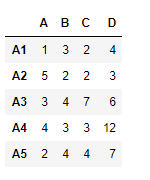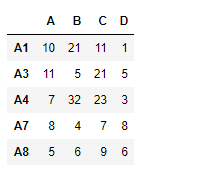Let’s use the dataframe.reindex_like() function to find the matching indexes.

 # find matching indexesdf1.reindex_like(df2)

Output :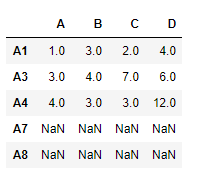Notice the output, unmatched indexes are populated with NaN values, we can fill in the missing values using ‘ffill’ method.

 # filling the missing values using ffill methoddf1.reindex_like(df2, method ='ffill')

Output :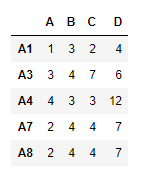Notice in the output, the new indexes has been populated using the “A5” row.

Example #2: Use reindex_like() function to match the indexes of two dataframes with limit on filling the missing values.

 # importing pandas as pdimport pandas as pd  # Creating the first dataframe df1 = pd.DataFrame({"A":[1, 5, 3, 4, 2],                    "B":[3, 2, 4, 3, 4],                    "C":[2, 2, 7, 3, 4],                    "D":[4, 3, 6, 12, 7]},                    index =["A1", "A2", "A3", "A4", "A5"])  # Creating the second dataframedf2 = pd.DataFrame({"A":[10, 11, 7, 8, 5],                    "B":[21, 5, 32, 4, 6],                    "F":[11, 21, 23, 7, 9],                    "K":[1, 5, 3, 8, 6]},                    index =["A1", "A2", "A3", "A4", "A7"])  # matching the indexesdf1.reindex_like(df2)

Output :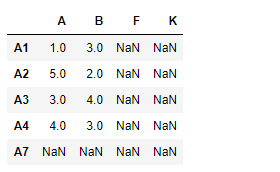Notice the output, unmatched indexes are populated with NaN values, we can fill in the missing values using ‘ffill’ method. we also limit the number of consecutive unmatched indexes that could be filled using the limit parameter.

 # match the indexes# fill the unmatched index using 'ffill' method# maximum consecutive unmatched indexes to be filled is 1  df.reindex_like(df1, method ='ffill', limit = 1)

Output :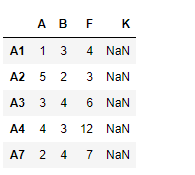My Personal Notes arrow_drop_up